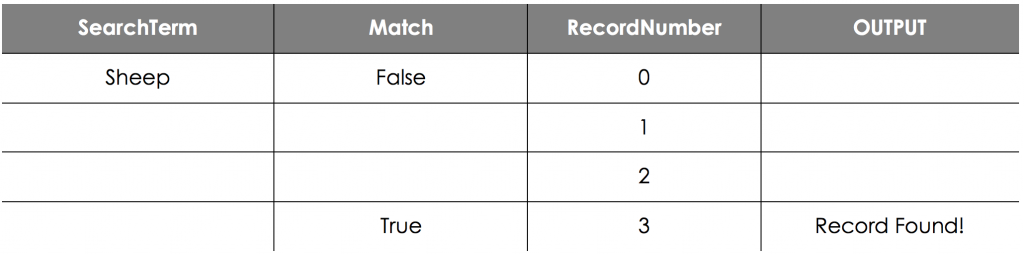Searching Algorithms

Searching Algorithms

A very common task that computers need to perform is searching for an item in a list. Many highly optimised search algorithms have been created, as companies like Google rely on effective searching algorithms all of the time. There are two basic searching algorithms that you need to be aware of:

1. Linear Search
2. Binary Search

The most basic search is called a linear search. You can think of a linear search as simply starting at the beginning of a list and checking each item until you find the thing you are looking for. It is the most natural way of looking for an item in a list, and you are likely to use it every day without realising it!

In general, simple algorithms tend to be rather inefficient - particularly when working with long arrays. This certainly applies with the linear search algorithm.

Here is an example of the linear search algorithm:

Arr ← [Item1, Item2, Item3, Item4]

SearchTerm ← USERINPUT

Match ← False

RecordNumber ← 0

REPEAT

IF Arr[RecordNumber] = SearchTerm THEN Match = True

RecordNumber ← RecordNumber + 1

UNTIL RecordNumber = LEN(Arr) OR Match = True

IF Match = True THEN

OUTPUT “Record found!”

ELSE

ENDIF

Let’s say we have this list:

[“Pig”, “Cat”, “Sheep”, “Dog”, “Cow”, “Horse”, “Donkey”, “Chicken”]

We want to check whether the value ‘Sheep’ is in the list so we run the algorithm, which gives us the trace table as follows:Even in just this short example, the program is not very efficient. We have to do 3 different checks before we find the value we want.

A binary search is a way of looking for a piece of data in an ordered list by continually splitting the list in half. You then check the middle number and work out which half of the list the value to find it.

The idea of a binary search is much like the way you might look through a phonebook for a phone number (the old-fashioned way!). We first look at the middle of the phonebook and see if the person’s name is there. If not, we work out whether they will be before that middle page or after it and then look at the middle of that section.

We keep repeating this until the person is found.

In simple terms, the algorithm can be described as follows:

1. Let StartPointer = 0 and EndPointer = n-1.
2. Compute MidPointer as the average of StartPointer and EndPointer, rounded down (so that it is an integer).
3. If array[MidPointer] equals SearchTerm, then stop.
4. If the value was too low, that is, array[MidPointer] < SearchTerm, then set StartPointer = MidPointer + 1.
5. Otherwise, the value was too high. Set EndPointer = MidPointer.
6. If StartPointer is equal to EndPointer, there are no more values to check, so stop.
7. Go back to step 2.
8. If array[MidPointer] equals SearchTerm then the value has been found, if not, it’s not in the list.

The pseudocode for this algorithm is significantly more difficult to follow than the linear search and there are lots of variations.

The simplest looks like this:

Arr ← [1, 3, 4, 6, 7, 8, 10, 13, 14]

SearchTerm ← 4

StartPointer ← 0

EndPointer ← LEN(Arr) - 1

REPEAT

MidPointer ← (StartPointer + EndPointer ) DIV 2 # round answer down

IF Record[MidPointer] < SearchTerm THEN

StartPointer ← MidPointer + 1

ELSE IF Record[MidPointer] > SearchTerm THEN

EndPointer ← MidPointer

ENDIF

UNTIL Record[MidPointer] = SearchTerm OR StartPointer = EndPointer

IF Record[MidPointer] = SearchTerm THEN

OUTPUT “Record found!”

ELSE